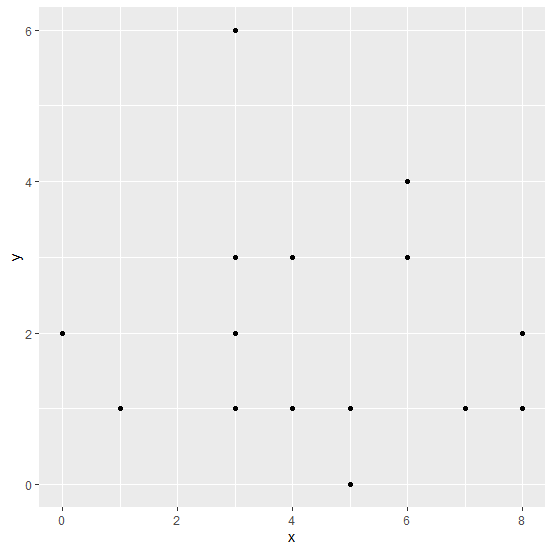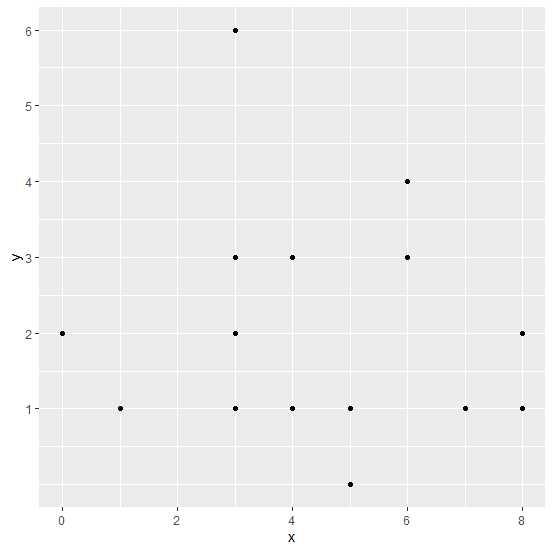# How to set the Y-axis tick marks using ggplot2 in R?

The default value of Y-axis tick marks using ggplot2 are taken by R using the provided data but we can set it by using scale_y_continuous function of ggplot2 package. For example, if we want to have values starting from 1 to 10 with a gap of 1 then we can use scale_y_continuous(breaks=seq(1,10,by=1)).

## Example

Live Demo

Consider the below data frame:
x<-rpois(20,5)
y<-rpois(20,2)
df<-data.frame(x,y)
df

## Output

   x y
1  8 1
2  4 3
3  1 1
4  4 1
5  3 2
6  3 6
7  6 4
8  3 3
9  7 1
10 4 1
11 5 1
12 6 3
13 3 1
14 6 3
15 8 2
16 0 2
17 8 1
18 5 0
19 4 1
20 4 3

Loading ggplot2 package and creating a point chart between x and y −

## Example

library(ggplot2)
ggplot(df,aes(x,y))+geom_point()

## OutputCreating the point chart with Y-axis tick marks with a difference of 1 −

## Example

ggplot(df,aes(x,y))+geom_point()+scale_y_continuous(breaks=seq(1,10,by=1))

## Output